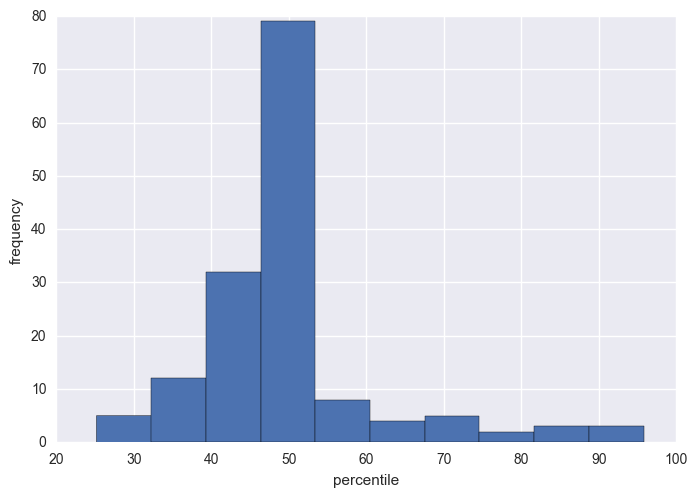KQ3: Pritts Meta-analysis Update¶

In key question 3, we address the risk of sarcoma dissemination following morcellation of fibroids. We identified a recently published analysis conducted by Elizabeth Pritts and her colleagues (2015) to estimate the prevalence of occult leiomyosarcoma at time of treatment for presumed benign tumors (fibroids). We updated their search and used similar eligibility criteria to identify papers published since 2014. We extracted from these papers the number of women who were treated for uterine fibroids and the cases of leiomyosarcoma subsequently identified. We have combined these data with the data from the 134 publications that Pritts et al included in their analysis for a total of LMS rates from 148 sources.

In :
%matplotlib inline
import numpy as np
import pandas as pd
import pymc3 as pm
import seaborn as sns
import matplotlib.pyplot as plt
import pdb
sns.set()

rseeds = 20090425, 19771114

In :
kq3_data = pd.read_excel('data/UF CER KQ3 Data for Analysis.xlsx', sheetname='5.UFKQ3Data',
na_values=['NR', 'NA'],
index_col=0)[['Author', 'Year', 'Design', 'Procedure', 'Indication',
'Age, Mean', 'Age, SD', 'Age, Median', 'Age, Min', 'Age, Max', 'Age, Other',
'LMS','Population','Tumors','InPritts']].dropna(thresh=7)

Out:
Author Year Design Procedure Indication Age, Mean Age, SD Age, Median Age, Min Age, Max Age, Other LMS Population Tumors InPritts
Line
1 Adelusola KA, Ogunniyi SO 2001 Retrospective NE NE NaN NaN NaN 19.0 89 NaN 0 177 0 1
2 Ahmed AA, Stachurski J, Abdel Aziz E et al 2002 Prospective NE NE NaN NaN NaN 29.0 65 NaN 0 10 0 1
3 Angle HS, Cohen SM, Hidlebaugh D 1995 Retrospective NE NE 41.0 NaN NaN 24.0 81 NaN 0 41 0 1
4 Banaczek Z, Sikora K, Lewandowska-Andruszuk I 2004 Retrospective NE NE 44.5 NaN NaN 29.0 73 NaN 0 309 0 1
5 Barbieri R, Dilena M, Chumas J, Rein MS, Fried... 1993 RCT NE NE 33.7 NaN NaN NaN NaN 36.3 0 20 0 1

Missing values

In :
kq3_data.isnull().sum()

Out:
Author           0
Year             0
Design           0
Procedure        0
Indication       3
Age, Mean       70
Age, SD        147
Age, Median    150
Age, Min        64
Age, Max        65
Age, Other     127
LMS              0
Population       0
Tumors           0
InPritts         0
dtype: int64
In :
kq3_data['age_max'] = kq3_data['Age, Max'].replace('50+', 50)
kq3_data = kq3_data.rename(columns={'Age, Min': 'age_min',
'Age, Mean': 'age_mean',
'Age, SD': 'age_sd',
'Age, Median': 'age_med'})

In :
kq3_data.describe()

Out:
Year age_mean age_sd age_med age_min LMS Population Tumors InPritts age_max
count 161.000000 91.000000 14.000000 11.000000 97.00000 161.000000 161.000000 161.000000 161.000000 96.000000
mean 2005.149068 42.117912 7.315000 41.545455 26.91134 2.652174 996.900621 2.652174 0.832298 61.466667
std 7.865440 6.413083 2.977962 5.791954 6.20752 16.441662 3927.579174 16.441662 0.374767 13.430732
min 1984.000000 28.200000 1.800000 35.000000 18.00000 0.000000 5.000000 0.000000 0.000000 34.000000
25% 1999.000000 37.320000 6.000000 37.450000 22.00000 0.000000 40.000000 0.000000 1.000000 51.000000
50% 2006.000000 42.500000 6.600000 40.000000 26.00000 0.000000 92.000000 0.000000 1.000000 60.000000
75% 2012.000000 46.000000 7.812500 45.800000 31.00000 0.000000 368.000000 0.000000 1.000000 70.250000
max 2016.000000 61.200000 12.500000 52.900000 44.00000 172.000000 34603.000000 172.000000 1.000000 96.000000
In :
kq3_data[[c for c in kq3_data.columns if c.startswith('age')]].hist();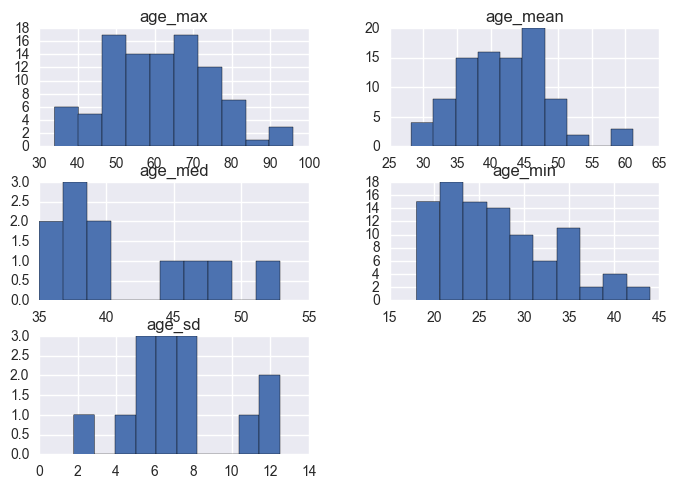Breakdown on studies by design.

In :
kq3_data['Design'] = kq3_data.Design.str.strip()

In :
kq3_data.Design.value_counts()

Out:
Retrospective                     88
Prospective                       38
RCT                               27
Retrospective Cohort               4
Pop based cohort                   1
Prospective cohort pilot study     1
Cohort                             1
Observational study                1
Name: Design, dtype: int64

I will restrict the analysis to the top three study designs.

In :
design_subset = ['Retrospective', 'Prospective', 'RCT']

In :
kq3_data = kq3_data[kq3_data.Design.isin(design_subset)]

In :
kq3_data = pd.concat([kq3_data, pd.get_dummies(kq3_data.Design)[['Prospective', 'RCT']]], axis=1)

In :
kq3_data['design_ind'] = kq3_data.Design.replace({'Retrospective':0, 'Prospective':1, 'RCT':2})

In :
kq3_data.loc[kq3_data.Design=='Prospective', ['Population','Tumors']]

Out:
Population Tumors
Line
2 10 0
6 91 0
7 75 0
9 24 0
10 25 0
13 80 0
14 136 0
15 11 0
18 25 0
21 9 0
27 213 0
28 71 0
31 17 0
44 47 0
46 89 0
50 75 0
52 28 0
54 25 0
55 45 0
56 368 0
57 8 0
63 167 0
64 486 0
69 80 0
73 69 0
80 331 0
85 30 0
89 38 0
91 8 0
108 37 0
110 505 2
115 235 0
119 92 1
120 9 0
122 51 0
123 18 0
125 6 0
127 5 0

Following Pritts et al. (2015), I fit a binomial random effects model, such that event probabilities on the logit scale are normally distributed with mean $\mu$ and standard deviation $\sigma$. This distribution describes how the probabilities vary across studies, with the degree of variation described by $\sigma$.

$$\theta_i \sim N(\mu, \sigma^2)$$

the expected value for study $i$ is then inverse-logit transformed, and used as the event probability $\pi_i$ in a binomial model describing the number of observed tumors $t$:

$$\log\left[\frac{\pi_i}{1-\pi_i}\right] = \theta_i$$$$t_i \sim \text{Bin}(n_i, \pi_i)$$
In :
import theano.tensor as tt

model_data = kq3_data

k = model_data.shape
tumors = model_data.Tumors.values.astype(int)
n = model_data.Population.values.astype(int)
X = model_data[['Prospective', 'RCT']].values.astype(int)
design = model_data.design_ind.values.astype(int)
age_max_norm = ((model_data.age_max - 60)/10).fillna(0.5).values

poly_terms = 3

invlogit = pm.math.invlogit

In :
with pm.Model() as pritts_update:

# Impute missing max ages
ν = pm.HalfCauchy('ν', 5, testval=1)
μ_age = pm.Normal('μ_age', 0, 5, testval=0)
age_max = pm.StudentT('age_max', ν, mu=μ_age, observed=age_max_missing)

# Study random effect
μ = pm.Normal('μ', 0, sd=100, testval=-3)
σ = pm.Uniform('σ', 0, 1000, testval=10)
θ = pm.Normal('θ', μ, sd=σ, shape=k)
# Design effects
β = pm.Normal('β', 0, sd=10, shape=2, testval=np.zeros(2))
# Age effect
α = pm.Normal('α', 0, sd=10, testval=0)

# Study-specific probabilities
π = pm.Deterministic('π', invlogit(θ + tt.dot(X,β) + α*age_max))

# Expected probabilities by design
p_retro_10000 = pm.Deterministic('p_retro_10000', invlogit(μ)*10000)
p_prosp_10000 = pm.Deterministic('p_prosp_10000', invlogit(μ + β)*10000)
p_rct_10000 = pm.Deterministic('p_rct_10000', invlogit(μ + β)*10000)

obs = pm.Binomial('obs', n=n, p=π, observed=tumors)

In :
with pritts_update:

Average ELBO = -308.35: 100%|██████████| 40000/40000 [00:10<00:00, 3764.53it/s]
Finished [100%]: Average ELBO = -305.43
100%|██████████| 3000/3000 [01:42<00:00, 29.32it/s]

In :
with pm.Model() as pritts_update_noage:

# Study random effect
μ = pm.Normal('μ', 0, sd=100, testval=-3)
σ = pm.Uniform('σ', 0, 1000, testval=10)
θ = pm.Normal('θ', μ, sd=σ, shape=k)
# Design effects
β = pm.Normal('β', 0, sd=10, shape=2, testval=np.zeros(2))

# Study-specific probabilities
π = pm.Deterministic('π', invlogit(θ + tt.dot(X,β)))

# Expected probabilities by design
p_retro_10000 = pm.Deterministic('p_retro_10000', invlogit(μ)*10000)
p_prosp_10000 = pm.Deterministic('p_prosp_10000', invlogit(μ + β)*10000)
p_rct_10000 = pm.Deterministic('p_rct_10000', invlogit(μ + β)*10000)

obs = pm.Binomial('obs', n=n, p=π, observed=tumors)

Applied interval-transform to σ and added transformed σ_interval_ to model.
/Users/fonnescj/Repositories/pymc3/pymc3/distributions/continuous.py:59: UserWarning: The variable specified for sd has non-positive support for Normal, likely making it unsuitable for this parameter.
warnings.warn(msg)

In :
with pritts_update_noage:
trace = pm.sample(2000, random_seed=rseeds)#, random_seed=[20140425, 19700903])

Assigned NUTS to μ
Assigned NUTS to σ_interval_
Assigned NUTS to θ
Assigned NUTS to β
100%|██████████| 2000/2000 [00:30<00:00, 66.39it/s]

In :
with pm.Model() as pritts_uncond:

# Impute missing max ages
ν = pm.HalfCauchy('ν', 5, testval=1)
μ_age = pm.Normal('μ_age', 0, 5, testval=0)
age_max = pm.StudentT('age_max', ν, mu=μ_age, observed=age_max_missing)

# Study random effect
μ = pm.Normal('μ', 0, sd=100, testval=-3)
σ = pm.Uniform('σ', 0, 1000, testval=10)
θ = pm.Normal('θ', μ, sd=σ, shape=k)
# Design effects
β = pm.Normal('β', 0, sd=10, shape=2, testval=np.zeros(2))
α = pm.Normal('α', 0, sd=10, testval=0)

# Study-specific probabilities
π = pm.Deterministic('π', invlogit(θ + α*age_max))

p_10000 = pm.Deterministic('p_10000', invlogit(μ)*10000)

obs = pm.Binomial('obs', n=n, p=π, observed=tumors)

Applied log-transform to ν and added transformed ν_log to model.
Applied interval-transform to σ and added transformed σ_interval to model.

In :
with pritts_uncond:
trace_uncond = pm.sample(2000, random_seed=rseeds)#, random_seed=[20140425, 19700903], njobs=2)

Assigned NUTS to ν_log_
Assigned NUTS to μ_age
Assigned NUTS to age_max_missing
Assigned NUTS to μ
Assigned NUTS to σ_interval_
Assigned NUTS to θ
Assigned NUTS to β
Assigned NUTS to α
[-----------------100%-----------------] 2000 of 2000 complete in 174.7 sec

The following plots are the distribution of samples from the posterior distributions for the expected (population) probability of tumor (p_update), the inverse-logit expected probability ($\mu$) and the standard deviation of the probabilities on the inverse-logit scale ($\sigma$).

In :
pm.traceplot(trace[-1000:], varnames=['p_retro_10000', 'p_prosp_10000', 'p_rct_10000',
'μ', 'σ']);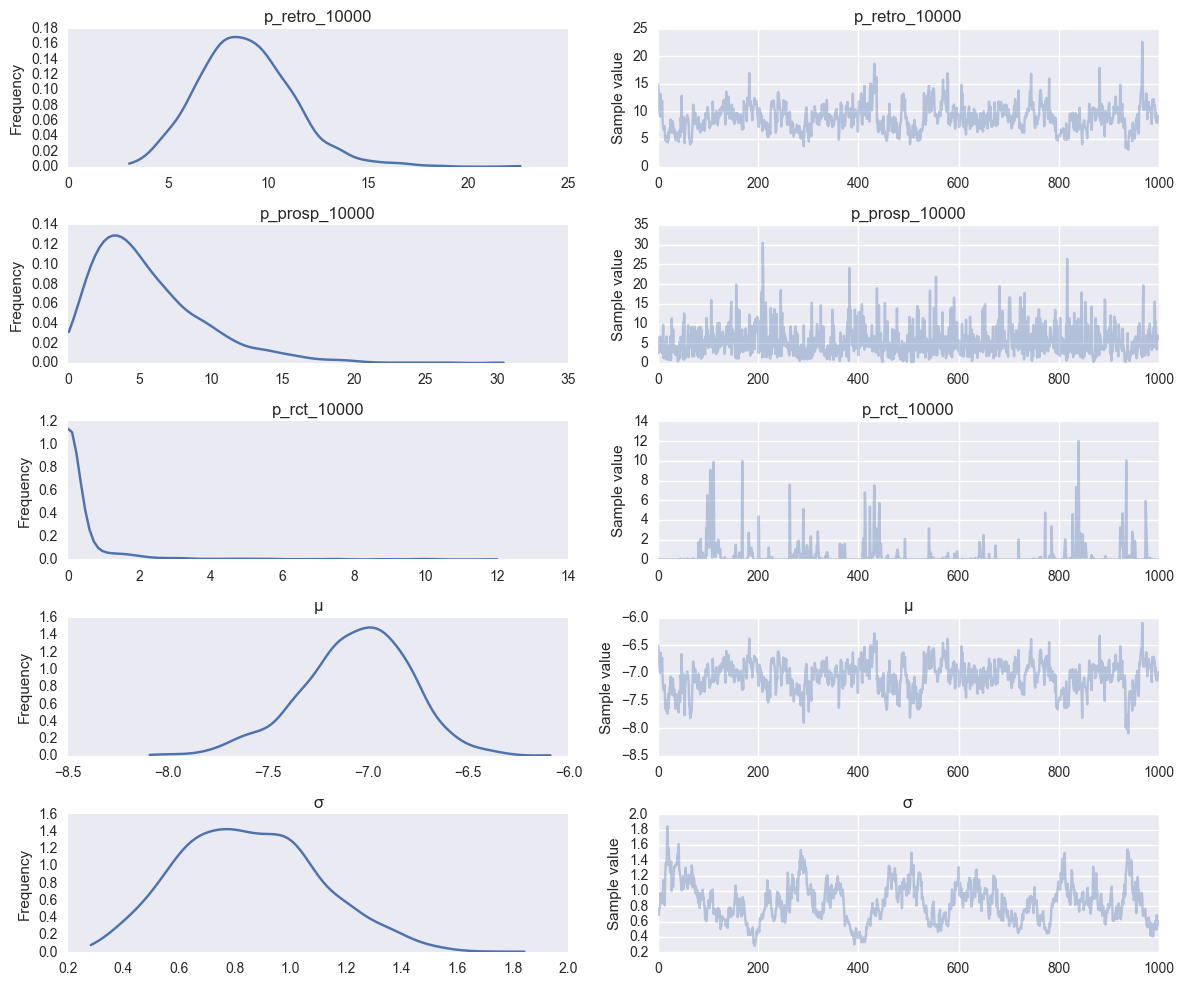Summary statistics from each of the above parameters.

In :
pm.summary(trace[-1000:], varnames=['p_retro_10000', 'p_prosp_10000', 'p_rct_10000', 'μ', 'σ'], roundto=4)

p_retro_10000:

Mean             SD               MC Error         95% HPD interval
-------------------------------------------------------------------

8.8856           2.3711           0.1747           [4.7012, 13.6712]

Posterior quantiles:
2.5            25             50             75             97.5
|--------------|==============|==============|--------------|

4.7732         7.3025         8.7350         10.2718        13.8700

p_prosp_10000:

Mean             SD               MC Error         95% HPD interval
-------------------------------------------------------------------

5.5972           3.9193           0.1593           [0.4361, 13.6899]

Posterior quantiles:
2.5            25             50             75             97.5
|--------------|==============|==============|--------------|

0.8226         2.8367         4.6300         7.4328         15.5504

p_rct_10000:

Mean             SD               MC Error         95% HPD interval
-------------------------------------------------------------------

0.3536           1.1564           0.0554           [0.0000, 1.8605]

Posterior quantiles:
2.5            25             50             75             97.5
|--------------|==============|==============|--------------|

0.0000         0.0005         0.0068         0.1107         3.3614

μ:

Mean             SD               MC Error         95% HPD interval
-------------------------------------------------------------------

-7.0610          0.2723           0.0205           [-7.6544, -6.5873]

Posterior quantiles:
2.5            25             50             75             97.5
|--------------|==============|==============|--------------|

-7.6468        -7.2214        -7.0421        -6.8799        -6.5792

σ:

Mean             SD               MC Error         95% HPD interval
-------------------------------------------------------------------

0.8534           0.2522           0.0226           [0.3870, 1.3387]

Posterior quantiles:
2.5            25             50             75             97.5
|--------------|==============|==============|--------------|

0.4058         0.6632         0.8425         1.0187         1.3874



By comparison, estimates from Pritts' supplement:In :
p_vars = ['p_retro_10000', 'p_prosp_10000', 'p_rct_10000']
intervals = [pm.stats.hpd(trace[var][-1000:]) for var in p_vars]

In :
meds = [np.median(trace[var][-1000:]) for var in p_vars]
upper = [i - m for m,i in zip(meds, intervals)]
lower = [m - i for m,i in zip(meds, intervals)]

plt.errorbar(np.arange(3)-0.1, meds, yerr=[lower, upper], fmt='s', label='Updated MA')
plt.errorbar(np.arange(2)+0.1, [5.7, 1.2], yerr=[[5.7-1.7, 1.2], [11.3 - 5.7,7.5 - 1.2]],
color='r', fmt='D', label='Pritts MA', elinewidth=0.5)
plt.xticks(range(3), ['Retrospective', 'Prospective', 'RCT'])
plt.ylabel('Rate per 10,000 surgeries')
plt.xlim(-0.5, 2.25)
plt.legend();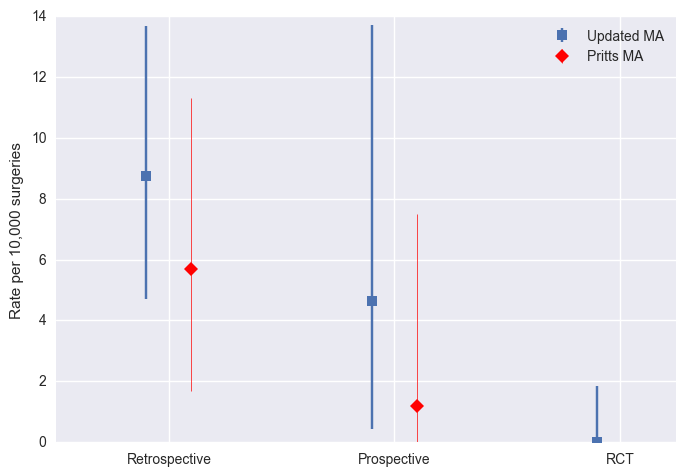In :
pm.forestplot(trace[1000:], varnames=['age_max_missing'], ylabels=[''])

Out:
<matplotlib.gridspec.GridSpec at 0x1219b4048>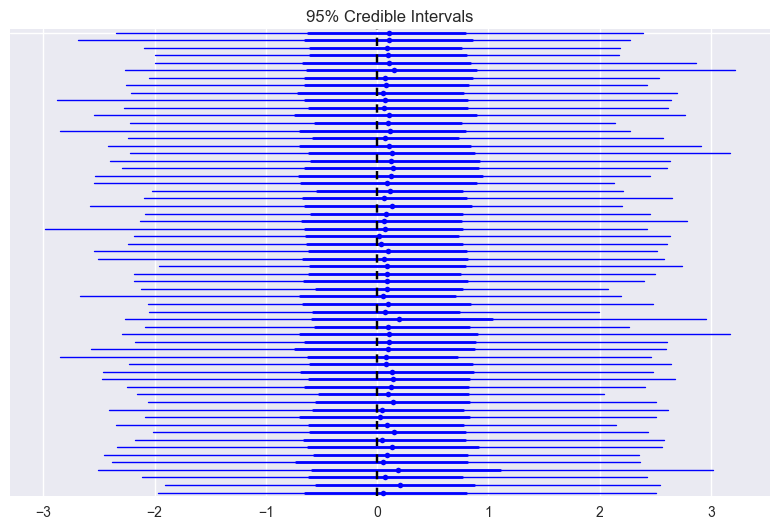Pooled estimates

In :
pm.traceplot(trace_uncond[1000:], varnames=['p_10000',
'α', 'μ', 'σ']);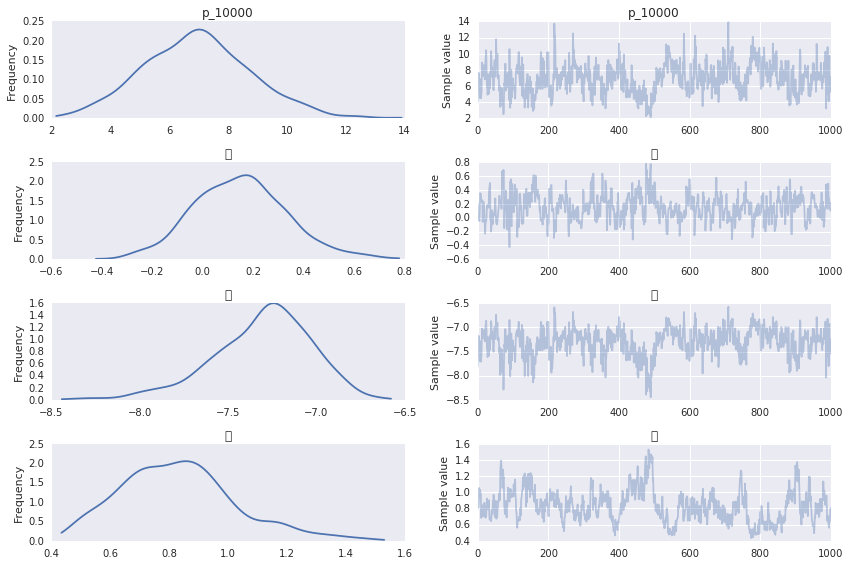In :
pm.summary(trace_uncond[1000:], varnames=['p_10000',
'α', 'μ', 'σ']);

p_10000:

Mean             SD               MC Error         95% HPD interval
-------------------------------------------------------------------

6.965            1.839            0.131            [3.196, 10.338]

Posterior quantiles:
2.5            25             50             75             97.5
|--------------|==============|==============|--------------|

3.496          5.677          6.944          8.132          10.769

α:

Mean             SD               MC Error         95% HPD interval
-------------------------------------------------------------------

0.149            0.184            0.011            [-0.242, 0.496]

Posterior quantiles:
2.5            25             50             75             97.5
|--------------|==============|==============|--------------|

-0.199         0.020          0.147          0.269          0.548

μ:

Mean             SD               MC Error         95% HPD interval
-------------------------------------------------------------------

-7.306           0.282            0.021            [-7.893, -6.811]

Posterior quantiles:
2.5            25             50             75             97.5
|--------------|==============|==============|--------------|

-7.958         -7.473         -7.272         -7.114         -6.833

σ:

Mean             SD               MC Error         95% HPD interval
-------------------------------------------------------------------

0.832            0.197            0.017            [0.474, 1.202]

Posterior quantiles:
2.5            25             50             75             97.5
|--------------|==============|==============|--------------|

0.494          0.692          0.820          0.941          1.284



Goodness of fit¶

To check how well the model fits the data, I conducted posteior predictive checks. This simulates data from the model for each data point (i.e. study) in the meta-analysis, and compares the distribution of these simulated values (here, 500 replicates) with the value of the data itself. If the percentile of the datum is very extreme (either large or small), then it is evidence that the model does not adequately fit the data. The distribution of percentiles in the bottom histogram shows that there is no evidence of lack of fit.

In :
ppc = pm.sample_ppc(trace, model=pritts_update, samples=500)

100%|██████████| 500/500 [00:07<00:00, 62.93it/s]

In :
from scipy.stats import percentileofscore

p = [percentileofscore(s, o).round(2) for s,o in zip(ppc['obs'].T, tumors)]

plt.hist(p)
plt.xlabel('percentile')
plt.ylabel('frequency');